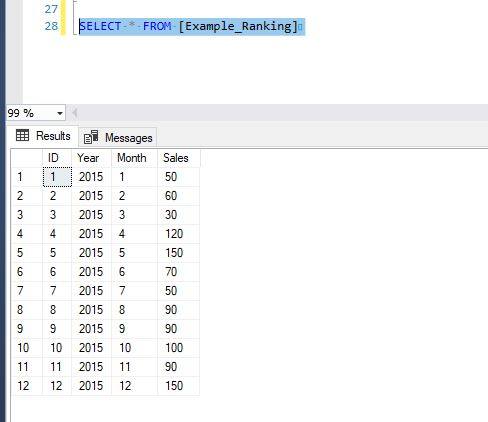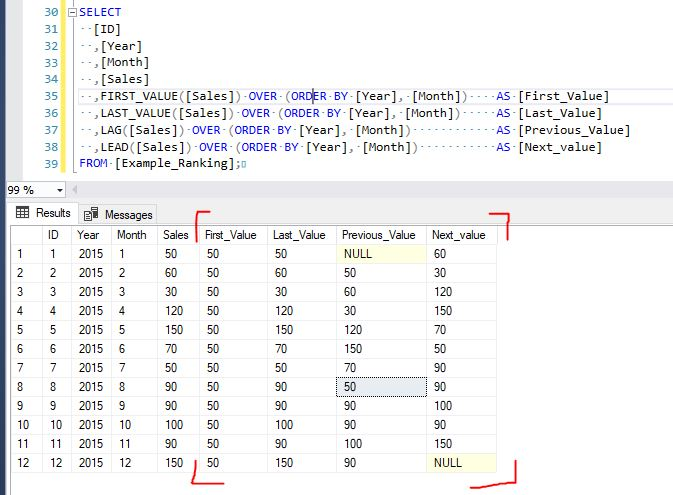• 13. 11. 2019
• Ing. Jan Zedníček - Data & Finance
• 0

OFFSET functions are relatively new to MS SQL Server. They are available since SQL Server 2012 version. These functions enable a user to “list” through rows of a table. To be precise, it makes you able to put hand on previous or next row while still at the current one.

These functions belong to a group of so-called Window functions. Aggregate functions and Ranking functions belong to the same group.

## List of SQL Functions For The Access to Previous or Next Rows

• LAG() – Finds the previous value in the table and returns it
• LEAD() – Finds the next value in the table and returns it
• FIRST_VALUE() – Finds and returns the lowest value according to the current row
• LAST_VALUE() – Finds and returns the highest value according to the current row

Another way how to page through a table is OFFSET <nr of rows> FETCH NEXT <nr of rows> command. This command is used with clause ORDER BY. However, this is not a function and therefore it does not belong here.

## Practical Example – LAG, LEAD, FIRST_VALUE, LAST_VALUE

We will create a table in which we will have 2015 Sales, month after month. We will then demonstrate offset functions on it. At the end we will try to write SQL script creating an index => month-on-month sales [%] using functions.

`USE [tempdb];`
`CREATE TABLE [Example_Ranking] (`
`  [ID] INT IDENTITY(1,1)`
`  ,[Year] INT`
`  ,[Month] INT`
`  ,[Sales] NUMERIC`
`);`

`INSERT INTO [Example_Ranking] (`
`  [Year]`
`  ,[Month]`
`  ,[Sales]`
`)`
`VALUES`
`(2015, 1, 50)`
`,(2015, 2, 60)`
`,(2015, 3, 30)`
`,(2015, 4, 120)`
`,(2015, 5, 150)`
`,(2015, 6, 70)`
`,(2015, 7, 50)`
`,(2015, 8, 90)`
`,(2015, 9, 90)`
`,(2015, 10, 100)`
`,(2015, 11, 90)`
`,(2015, 12, 150)`

The table looks like this:Script:

`SELECT`
`  [ID]`
`  ,[Year]`
`  ,[Month]`
`  ,[Sales]`
`  ,FIRST_VALUE([Sales]) OVER (ORDER BY [Year], [Month])    AS [First_Value]`
`  ,LAST_VALUE([Sales]) OVER (ORDER BY [Year], [Month])     AS [Last_Value]`
`  ,LAG([Sales]) OVER (ORDER BY [Year], [Month])            AS [Previous_Value]`
`  ,LEAD([Sales]) OVER (ORDER BY [Year], [Month])           AS [Next_value]`
`FROM [Example_Ranking];`

Result:## How to Make an Index (month-on-month change) Using LAG() Function?

We can count a bunch of stuff using offset functions – for example, rate of growth between members of time line i.e. index. There is an example how to do it:

`SELECT`
`  [ID]`
`  ,[Year]`
`  ,[Month]`
`  ,LAG([Sales]) OVER (ORDER BY [Year], [Month])         AS [Previous_Mesic]`
`  ,[Sales]                                              AS [Actual_mesic]`
`  ,CONVERT(NUMERIC(10,2),`
`      100*([Sales] - LAG([Sales]) OVER (ORDER BY [Year], [Month]))`
`        /`
`      LAG([Sales]) OVER (ORDER BY [Year], [Month]))     AS [Index %]`
`FROM [Example_Ranking];`

Result:Rate this post### Ing. Jan Zedníček - Data & Finance

My name is Jan Zedníček and I work as a freelancer. I have been working as a freelancer for many companies for more than 10 years.
I used to work as a financial controller, analyst and manager at many different companies in field of banking and manufacturing. When I am not at work, I like playing volleyball, chess, doing a workout in the gym.